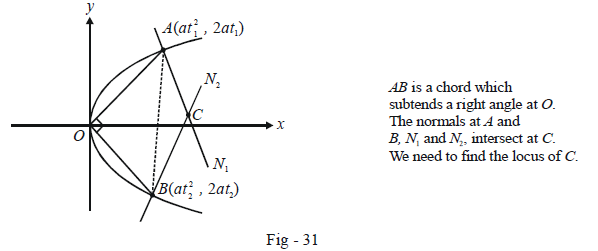# Examples on Normals To Parabolas Set 2

Go back to  'Parabola'

Example - 29

Find the locus of the point of intersection of normals drawn to the parabola $${y^2} = 4ax$$ at the extremities of a chord which subtends a right angle at the vertex of the parabola.

Solution: Let $$A(at_1^2,\,2a{t_1}){\rm{ }}\;and\;{\rm{ }}B(at_2^2,\,2a{t_2})$$ be the extremities of a chord which subtends a right angle at the vertex (0, 0);Since $$OA \bot OB,$$ we have

\begin{align}& \qquad \underbrace {\left( {\frac{{2a{t_1} - 0}}{{at_1^2 - 0}}} \right)}_{{\rm{slope\;of \;}}OA} \times \underbrace {\left( {\frac{{2a{t_2} - 0}}{{at_2^2 - 0}}} \right)}_{{\rm{slope\;of\;}}OB} = - 1\\&\Rightarrow \qquad {t_1}{t_2} = - 4\end{align}

The equation to $${N_1}\;and\;{\rm{ }}{N_2}$$ can be written using the standard form of a normal at a point t :

\begin{align}&{N_1}:y + {t_1}x = 2a{t_1} + at_1^3\\\\&{N_2}:y + {t_2}x = 2a{t_2} + at_2^3\end{align}

Let the intersection of $${N_1}\,and\,{\rm{ }}{N_2}$$ be the point C(h, k). The co-ordinates of C can be evaluated by solving the equations of $${N_1}and{\rm{ }}{N_2}$$ simultaneously :

\begin{align}h &= 2a + a(t_1^2 + t_2^2 + {t_1}{t_2})\\\\&= - 2a + a(t_1^2 + t_2^2)\\\\\rm{and} \qquad {\rm{ }}k &= - a{t_1}{t_2}({t_1} + {t_2})\\\\&= 4a({t_1} + {t_2})\end{align}

We thus have, by eliminating $${t_1}\,and\,{\rm{ }}{t_2}$$ , a relation in h and k:

\begin{align}\frac{{{k^2}}}{{16{a^2}}} &= \frac{{h + 2a}}{a} - 8\\\\\Rightarrow \qquad {k^2} &= 16a(h - 6a)\end{align}

Using (x, y) instead of (h, k), the required locus is

${y^2} = 16a(x - 6a)$

Example – 30

Find the locus of the point of intersection of the three normals to the parabola $${y^2} = 4ax,$$ two of which are inclined at right angles to each other.

Solution: Let P(h, k) be the point whose locus we wish to determine. Any normal to the given parabola can be written as

$y = mx - 2am - a{m^3}$

If this passes through P(h, k), we have

$\;\;\quad k = mh - 2am - a{m^3} \qquad \dots \left( 1 \right)$

Let $${m_1},{\rm{ }}{m_2}{\rm{ }}\;and\;{\rm{ }}{m_3}$$ be the three roots of this cubic. It is given that two of the normals are perpendicular, implying that the product of two of these three slopes, say $${m_1}\;and\;{\rm{ }}{m_2}$$ is –1, i.e.$${m_1}{m_2}{\rm{ }} = {\rm{ }}-1.$$

From (1), we have

\begin{align}{m_1}{m_2}{m_3} &= \frac{{ - k}}{a}\\\\\Rightarrow \qquad {m_3} &= \frac{k}{a}\end{align}

Substituting this value of $${m_3}$$ back in (1), we obtain a relation between h and k :

\begin{align}& \qquad \;\; k = \frac{{hk}}{a} - 2k - \frac{{a{k^3}}}{{{a^3}}}\\\\&\Rightarrow \quad k({k^2} + (3a - h)a) = 0\end{align}

Using (x, y) instead of (h, k) the required locus is

$y({y^2} + (3a - x)a) = 0$

## TRY YOURSELF - III

 Q. 1 Find the equation of the normal to $${y^2} = 4x$$ which is perpendicular to $$2x + 6y + 5 = 0$$ . Q. 2 The normal at any point P on the parabola $${y^2} = 4ax$$ meets its axis in A and the y-axis in B. Let O be the origin. The rectangle OACB is completed. Find the locus of C. Q. 3 Prove that the normal to $${y^2} = 4ax$$ at a non-zero point whose x and y coordinates are equal, subtends a right angle at the focus. Q. 4 Prove that the normal at any point to a parabola is equally inclined to the focal chord passing through that point and the axis of the parabola. Q. 5 Have you ever heard of parabolic mirrors being very effective in concentrating incident light energy at a particular a point ? Can you think of a reason ? What would that particular point be ?